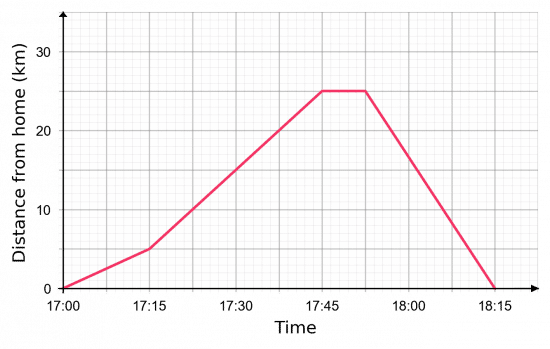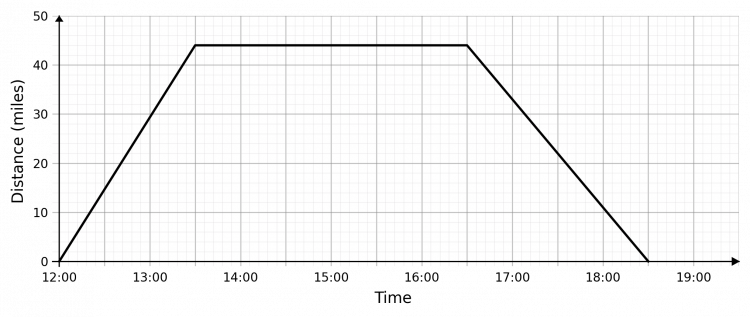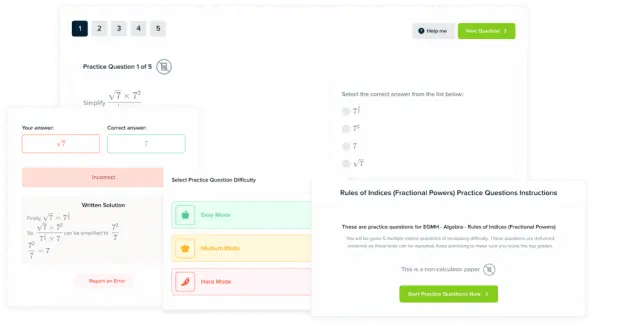# Distance-Time Graphs

GCSEKS3Level 4-5AQACambridge iGCSEEdexcelEdexcel iGCSEOCRWJEC

## Distance-Time Graphs

Distance-time graphs are a way of visually expressing a journey. With distance on the $y$-axis and time on the $x$-axis, a distance-time graph tells us how far someone/something has travelled and how long it took them/it to do so.

Make sure you are happy with the following topics before continuing:

Level 4-5GCSEKS3AQAEdexcelOCRWJECCambridge iGCSEEdexcel iGCSE

## Distance time graphs –  Key things to remember:

1) The gradient of the line = speed

2) A flat section means no speed (stopped)

3) The steeper the graph the greater the speed

4) Negative gradient = returning to start point (coming back)Level 4-5GCSEKS3AQAEdexcelOCRWJECCambridge iGCSEEdexcel iGCSE

## Using Distance Time Graphs

The graph below describes a journey that has several parts to it, each represented by a different straight line.

Part A: $\bf{09:00 - 11:00}$, the person travelled $30$ km away from their starting point and that took them $2$ hours.

Part B: $\bf{11:00 - 12:00}$, we can see that the line is flat, so the distance from their starting point did not change – they were stationary.

Part C: $\bf{12:00 - 12:30}$, they moved a further $30$ km away from their starting point.

Part D: $\bf{12:30 - 14:00}$, they travelled the full $60$ km back to where they began.Calculate the speed – for each part of the journey:

$\text{Speed} (S) = \dfrac{\text{Distance}(D)}{\text{Time}(T)}$

Part A: $\bf{09:00 - 11:00}$

$\text{Speed}= \dfrac{30}{2} = 15$ km/h

Part B: $\bf{11:00 - 12:00}$

$\text{Speed}= \dfrac{0}{1} = 0$ km/h (Not moving)

Part C: $\bf{12:00 - 12:30}$

$\text{Speed}= \dfrac{30}{0.5} = 60$ km/h

Part D: $\bf{12:30 - 14:00}$

$\text{Speed}= \dfrac{60}{1.5} = 40$ km/h

From this we can see that the person travelled the fastest over part C.

Level 4-5GCSEKS3AQAEdexcelOCRWJECCambridge iGCSEEdexcel iGCSE
Level 4-5GCSEKS3AQAEdexcelOCRWJECCambridge iGCSEEdexcel iGCSE

## Example: Bike Ride

Valentina is going for a bike ride. Below is a distance-time graph that describes her full journey.

a) How long was she stationary for?

b) What was the total distance travelled during her journey?

c) What was her average speed in kilometres per hour between $17:15$ and $17:45$?

[3 marks]a) We can see that the graph was flat for the duration of one big square. From the axis, we can see that two big squares total $15$ minutes, therefore one big square is worth $7.5$ minutes, so she was stationary for $7.5$ minutes.

b) Valentina travelled away $25$ km away from home, stopped briefly, and then travelled $25$ km back home. Therefore, she travelled $50$ km in total.

c) We need to calculate the gradient of the graph between $17:15$ and $17:45$. This period lasted for $30$ minutes, which is equivalent to $0.5$ hours – this is the “change in $x$”. During this period, she increased her distance from home from $5$ km up to $25$ km, meaning she travelled $20$ km in total – this is the “change in $y$”. So, we get

$\text{Gradient } = \dfrac{20}{0.5} = 40$ km/h

Level 4-5GCSEKS3AQAEdexcelOCRWJECCambridge iGCSEEdexcel iGCSE

## Distance-Time Graphs Example Questions

So, making the words above seem more readable, we get:

• $12:00 - 13:30$, he travels from $0$ miles away to $44$ miles away;
• $13:30 - 16:30$, he stays in one place;
• $16:30 - 18:30$, he travels from $44$ miles away to $0$ miles away.

On a graph, this looks like:Gold Standard Education

Looking at the graph, we can see that he runs at three different speeds during different portions of the race. The graph becomes less steep in the middle, so that won’t be his period of maximum speed, and the other two are hard to distinguish just by looking so we’ll work them both out.

Period 1:

$\text{Gradient } = \dfrac{\text{distance travelled}}{\text{time taken}} = \dfrac{600 - 0}{72 - 0} = 8.33$ m/s

Period 3:

$\text{Gradient } = \dfrac{\text{distance travelled}}{\text{time taken}} = \dfrac{1,500 - 880}{282 - 180} = 6.08$ m/s

Therefore, the fastest speed travelled by Chris during the race was $8.33$ m/s, to $3$ sf.Gold Standard Education

(a) Distance travelled is,

$\text{Total Distance } = 48 + 10 = 58$ km

(b) She stopped for $30$ mins at the $32$ km mark.Gold Standard Education

The gradient of a distance time graph is the speed. Hence to find the fastest average speed we must find the steepest section of the graph.

This is the final section which cover $48$ km in one hour, thus,

$\text{Maximum speed } = 48$ km/hGold Standard Education

## Distance-Time Graphs Worksheet and Example Questions

### (NEW) Distance Time Graphs Exam Style Questions - MME

Level 4-5GCSENewOfficial MME

## Distance-Time Graphs Drill Questions

### Distance Time Graphs - Drill Questions

Level 4-5GCSEProduct

### £19.99

/month

Learn an entire GCSE course for maths, English and science on the most comprehensive online learning platform. With revision explainer videos & notes, practice questions, topic tests and full mock exams for each topic on every course, it’s easy to Learn and Revise with the MME Learning Portal.

Level 4-5GCSEKS3

Level 1-3GCSEKS3

Level 4-5GCSEKS3